American Journal of Applied Mathematics
Volume 3, Issue 4, August 2015, Pages: 185-188

A Study on (Q,L)-Fuzzy Normal Subsemiring of a Semiring

S. Sampathu1, S. Anita Shanthi2, A. Praveen Prakash3

1Department of Mathematics, Sri Muthukumaran College of Education, Chikkarayapuram, Chennai, Tamil Nadu, India

2Department of Mathematics, Annamalai University, Tamil Nadu, India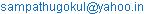(S. Sampathu)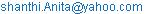(S. A. Shanthi)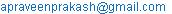(A. P. Prakash)

S. Sampathu, S. Anita Shanthi, A. Praveen Prakash. A Study on (Q,L)-Fuzzy Normal Subsemiring of a Semiring. American Journal of Applied Mathematics. Vol. 3, No. 4, 2015, pp. 185-188. doi: 10.11648/j.ajam.20150304.14

Abstract: In this paper, we introduce the concept of (Q,L)-fuzzy normal subsemirings of a semiring and establish some results on these. We also made an attempt to study the properties of (Q,L)-fuzzy normal subsemirings of semiring under homomorphism and anti-homomorphism , and study the main theorem for this. We shall also give new results on this subject.

Keywords: (Q,L)-Fuzzy Subset, (Q,L)-Fuzzy Subsemiring, (Q,L)-Fuzzy Normal Subsemiring, Product Of (Q,L)-Fuzzy Subsets, Strongest (Q, L)-Fuzzy Relation, Pseudo (Q, L)-Fuzzy Coset

Contents

1. Introduction

There are many concepts of universal algebras generalizing an associative ring ( R ;+; . ). Some of them in particular, nearrings and several kinds of semirings have been proven very useful. An algebra (R ; +, .) is said to be a semiring if (R;+) and (R; .) are semigroups satisfying a.(b+c)=a.b+a.c and (b+c).a=b.a+c.a for all a, b and c in R. A semiring R is said to be additively commutative if a+b = b+a for all a, b in R. A semiring R may have an identity 1, defined by 1. a = a = a. 1 and a zero 0, defined by 0+a=a=a+0 and a.0=0=0.a for all a in R. After the introduction of fuzzy sets by L.A.Zadeh, several researchers explored on the generalization of the concept of fuzzy sets. The notion of fuzzy subnearrings and ideals was introduced by S.Abou Zaid. A.Solairaju and R.Nagarajan  have introduced and defined a new algebraic structure called Q-fuzzy subgroups. In this paper, we introduce the concept of (Q,L)-fuzzy normal subsemiring of a semiring and established some results.

2. Preliminaries

2.1. Definition 1

Let X be a non-empty set and L = (L, ≤) be a lattice with least element 0 and greatest element 1 and Q be a non-empty set. A (Q, L)-fuzzy subset A of X is a function A: X×Q → L.

2.2. Definition 2

Let ( R, +, ∙ ) be a semiring and Q be a non empty set. A (Q, L)-fuzzy subset A of R is said to be a (Q, L)-fuzzy subsemiring (QLFSSR) of R if the following conditions are satisfied:

(i) A( x+y, q ) ≥ A(x, q) ˄ A(y, q),

(ii) A( xy, q ) ≥ A(x, q) ˄ A(y, q), for all x and y in R and q in Q.

2.3. Definition 3

Let R be a semiring and Q be a non-empty set. An (Q, L)-fuzzy subsemiring A of R is said to be an (Q, L)-fuzzy normal subsemiring (QLFNSSR) of R if it satisfies the following conditions:

(i)         A(x+y,q) = A(y+x,q),

(ii)       A(xy,q) = A(yx,q), for all x and y in R and q in Q.

2.4. Definition 4

Let A and B be any two (Q,L)-fuzzy subsets of sets G and H, respectively. The product of A and B, denoted by A×B, is defined as A×B={<(( x, y), q),A×B((x,y),q)>/ for all x in R and y in H and q in Q}, where A×B((x,y),q)=A(x, q)˄ B(y, q).

2.5. Definition 5

Let (R,+,∙ ) and (R׀,+,∙ ) be any two semirings and Q be a non empty set. Let f:R→R׀ be any function and A be a (Q,L)-fuzzy subsemiring in R, V be a (Q,L)-fuzzy subsemiring in f(R)=R׀, defined by V(y,q) =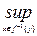A(x,q), for all x in R and y in R׀ and q in Q. Then A is called a pre-image of V under f and is denoted by f -1(V).

2.6. Definition 6

Let A be a (Q,L)-fuzzy subset in a set S, the strongest (Q, L)-fuzzy relation on S, that is a (Q,L)-fuzzy relation V with respect to A given by V((x,y),q) = A(x,q)˄A(y,q), for all x and y in S and q in Q.

2.7. Definition 7

A (Q,L)-fuzzy subset A of a set X is said to be normalized if there exists an element x in X such that A(x,q)=1.

2.8. Definition 8

Let A be an (Q, L)-fuzzy subsemiring of a semiring (R, +, ∙ ) and a in R. Then the pseudo (Q, L)-fuzzy coset (aA)p is defined by ( (aA)p)(x,q) = p(a)A(x,q), for every x in R and for some p in P and q in Q.

2.9. Definition 9

Let A be a (Q,L)-fuzzy subset of X. For a in L, a Q-level subset of A is the set Aa = { xÎX : A(x,q) ≥ a}.

3. Properties of (Q,L)-Fuzzy Normal Subsemiring of a Semiring

3.1. Theorem 1

Let (R,+,.) be a semiring and Q be a non-empty set. If A and B are two (Q,L)-fuzzy normal subsemirings of R, then their intersection A∩B is an (Q,L)-fuzzy normal subsemiring of R.

Proof: Let x and yÎR. Let A={á(x,q),A(x,q)ñ/ x in R and q in Q} and B={á(x,q), B(x,q)ñ/ x in R and q in Q} be (Q,L)-fuzzy normal subsemirings of a semiring R. Let C=A∩B and C={á(x,q),C(x,q)ñ/x in R and q in Q}. Then, Clearly C is an (Q,L)-fuzzy subsemiring of a semiring R, since A and B are two (Q,L)-fuzzy subsemirings of a semiring R. And (i) C(x+y,q)=A(x+y,q)˄B(x+y,q)=A(y+x,q)˄B(y+x,q) =C(y+x,q), for all x and y in R and q in Q.   Therefore,C(x+y,q) =(y+x,q), for all x and y in R and q in Q. (ii) C(xy,q) = A(xy,q)˄B(xy,q)=A(yx,q)˄B(yx,q)=C(yx,q), for all x and y in R and q in Q. Therefore, C(xy,q)=C(yx,q), for all x and y in R and q in Q. Hence A∩B is an (Q,L)-fuzzy normal subsemiring of a semiring R.

3.2. Theorem 2

Let R be a semiring and Q be a non-empty set. The intersection of a family of (Q,L)-fuzzy normal subsemirings of R is an (Q,L)-fuzzy normal subsemiring of R.

Proof: Let {Ai}iÎI be a family of (Q,L)-fuzzy normal subsemirings of a semiring R and let A=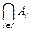. Then for x and y in R and q in Q. Clearly the intersection of a family of (Q,L)-fuzzy subsemirings of a semiring R is an (Q,L)-fuzzy subsemiring of a semiring R.(i) A(x+y,q) =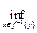Ai(x+y,q)=Ai(y+x,q)=A(y+x,q). Therefore, A(x+y,q)=A(y+x,q), for all x and y in R and q in Q. (ii) A(xy,q)=Ai(xy,q)=Ai(yx,q)=A(yx,q). Therefore, A(xy,q)=A(yx,q), for all x and y in R and q in Q.Hence the intersection of a family of (Q,L)-fuzzy normal subsemirings of a semiring R is an (Q,L)-fuzzy normal subsemiring of a semiring R.

3.3. Theorem 3

Let A and B be (Q,L)-fuzzy subsemiring of the semirings G and H, respectively. If A and B are (Q,L)-fuzzy normal subsemirings, then A×B is an (Q,L)- fuzzy normal subsemiring of G×H.

Proof: Let A and B be (Q,L)-fuzzy normal subsemirings of the semirings G and H respectively. Clearly A×B is an (Q,L)-fuzzy subsemiring of G×H. Let x1 and x2 be in G, y1 and y2 be in H and q in Q. Then (x1,y1) and (x2,y2) are in G×H. Now,A×B[(x1,y1)+(x2,y2),q]=A×B((x1+x2,y1+y2),q) =A(x1+x2,q)˄B(y1+y2,q)=A(x2+x1,q)˄B(y2+y1,q) =A×B((x2+x1,y2+y1),q)=A×B[(x2,y2)+(x1,y1),q]. Therefore,A×B[(x1,y1)+(x2,y2),q]=A×B[(x2,y2)+(x1,y1),q]. And,A×B[(x1,y1)(x2,y2),q]=A×B((x1x2,y1y2),q) =A(x1x2,q)˄B(y1y2,q)=A(x2x1,q),B(y2y1,q)= A×B((x2x1,y2y1),q)=A×B[(x2,y2)(x1,y1),q]. Therefore, A×B[(x1,y1)(x2,y2),q]=A×B[(x2,y2)(x1,y1),q]. Hence A×B is an (Q,L)-fuzzy normal subsemiring of G×H.

3.4. Theorem 4

Let A be a fuzzy subset in a semiring R and V be the strongest (Q,L)-fuzzy relation on R. Then A is an (Q,L)-fuzzy normal subsemiring of R if and only if V is an (Q,L)-fuzzy normal subsemiring of R×R.

Proof: Suppose that A is a (Q,L)-fuzzy normal subsemiring of R. Then for any x=(x1,x2) and y=(y1,y2) are in R×R and q in Q. Clearly V is a (Q,L)-fuzzy subsemiring of R×R. We have, V(x+y,q)=V[(x1,x2)+(y1,y2),q]=V((x1+y1,x2+y2),q) =A((x1+y1),q)ÙA((x2+y2),q)=A((y1+x1),q)ÙA((y2+x2),q) =V((y1+x1,y2+x2),q)=V[(y1,y2)+(x1,x2),q]=V(y+x,q) Therefore,V(x+y,q)=V(y+x,q), for all x and y in R×R and q in Q. We have, V(xy,q)=V[(x1,x2)(y1,y2),q]=V((x1y1,x2y2),q) =A((x1y1),q)ÙA((x2y2),q)=A((y1x1),q)ÙA((y2x2),q) =V((y1x1,y2x2),q)=V[(y1,y2)(x1,x2),q]=V(yx,q)       Therefore, V(xy,q)=V(yx,q), for all x and y in R×R and q in Q. This proves that V is a (Q,L)-fuzzy normal subsemiring of R×R. Conversely, assume that V is a (Q,L)-fuzzy normal subsemiring of R×R, then for any x=(x1,x2) and y=(y1,y2) are in R×R,we have    A(x1+y1,q)ÙA(x2+y2,q)=V((x1+y1,x2+y2),q) =V[(x1,x2)+(y1,y2),q]=V(x+y,q)=V(y+x,q) =V[(y1,y2)+(x1,x2),q]=V((y1+x1,y2+x2),q) =A(y1+x1,q)ÙA(y2+x2,q). We get, A((x1+y1),q)=A((y1+ x1),q), for all x1 and y1 in R and q in Q. And A(x1y1,q)ÙA(x2y2,q)=V((x1y1,x2y2),q)=V[(x1,x2)(y1,y2),q] =V(xy,q)=V(yx,q)=V[(y1,y2)(x1,x2),q]=V((y1x1,y2x2),q) =A(y1x1,q)Ù A(y2x2,q). We get, A(( x1y1),q)=A((y1x1),q), for all x1 and y1 in R and q in Q. Hence A is a (Q, L)-fuzzy normal subsemiring of R.

3.5. Theorem 5

Let (R,+, .) and (R׀,+, .) be any two semirings and Q be a non-empty set. The homomorphic image of an (Q,L)-fuzzy normal subsemiring of R is an (Q,L)-fuzzy normal subsemiring of R׀.

Proof: Let (R,+,.) and (R׀,+,.) be any two semirings Q be a non-empty set and f :R®R׀ be a homomorphism. Then, f(x+y)=f(x)+f(y) and f(xy)=f(x)f(y), for all x and y in R. Let V=f(A), where A is an (Q,L)-fuzzy normal subsemiring of a semiring R. We have to prove that V is an (Q,L)-fuzzy normal subsemiring of a semiring R׀. Now, for f(x), f(y) in R׀, clearly V is an (Q,L)-fuzzy subsemiring of a semiring R׀, since A is an (Q,L)-fuzzy subsemiring of a semiring R. Now, V(f(x)+f(y),q)=V(f(x+y),q)≥A(x+y,q)=A(y+x,q)≤V(f(y+x),q)=V(f(y)+f(x),q), which implies that V(f(x)+f(y),q)= V(f(y)+(f(x),q), for all f(x) and f(y) in R׀. Again, V(f(x)f(y),q)=V(f(xy),q)≥A(xy,q)=A(yx,q)≤V(f(yx),q) =V(f(y)f(x),q), which implies that V(f(x)f(y),q)=V(f(y)f(x),q ), for all f(x) and f(y) in R׀. Hence V is an (Q,L)-fuzzy normal subsemiring of a semiring R׀.

3.6. Theorem 6

Let (R,+,.) and (R׀,+,.) be any two semirings and Q be a non-empty set. The homomorphic preimage of an (Q,L)-fuzzy normal subsemiring of R׀ is an (Q,L)-fuzzy normal subsemiring of R.

Proof: Let ( R, +, .) and ( R׀, +, .) be any two semirings and Q be a non-empty set and f : R ® R׀ be a homomorphism. Then, f(x+y)=f(x)+f(y) and f(xy)=f(x)f(y), for all x and y in R. Let V=f(A), where V is an (Q,L)-fuzzy normal subsemiring of a semiring R׀. We have to prove that A is an (Q,L)-fuzzy normal subsemirring of a semiring R. Let x and y in R. Then, clearly A is an (Q,L)-fuzzy subsemiring of a semiring R, since V is an (Q,L)-fuzzy subsemiring of a semiring R׀. Now, A(x+y,q)=V(f(x+y),q)=V(f(x)+f(y),q)=V(f(y)+f(x),q)= V(f(y+x),q)=A(y+x,q), which implies that A(x+y,q)=A(y+x,q), for all x and y in R and q in Q. Again, A(xy,q)=V(f(xy),q)=V(f(x)f(y),q)=V(f(y)f(x),q)=V(f(yx),q)=A(yx,q), which implies that A(xy,q)= A(yx,q), for all x and y in R and q in Q. Hence A is an (Q,L)-fuzzy normal subsemiring of a semiring R.

3.7. Theorem 7

Let (R, +, .) and (R׀, +, .) be any two semirings and Q be a non-empty set. The anti-homomorphic image of an (Q, L)-fuzzy normal subsemiring of R is an (Q, L)-fuzzy normal subsemiring of R׀.

Proof: Let (R,+,.) and (R׀,+,.) be any two semirings and Q be a non-empty set and f:R®R׀ be an anti-homomorphism. Then, f(x+y)=f(y)+f(x) and f(xy)=f(y) f(x), for all x and y in R. Let V=f(A), where A is an (Q,L)-fuzzy normal subsemiring of a semiring R. We have to prove that V is an (Q,L)-fuzzy normal subsemiring of a semiring R׀. Now, for f(x) and f(y) in R׀, clearly V is an (Q,L)-fuzzy subsemiring of a semiring R׀, since A is an (Q,L)-fuzzy subsemiring of a semiring R. Now, V(f(x)+f(y),q)=V(f(y+x),q)≥A(y+x,q)=A(x+y,q)≤V(f(x+y),q)=V(f(y)+f(x),q), which implies that V(f(x)+f(y),q)=V(f(y)+f(x),q), for all f(x) and f(y) in R׀. Again,V(f(x)f(y),q)=V(f(yx),q)≥A(yx,q)=A(xy,q)≤V(f(xy),q)=V(f(y)f(x),q), which implies that V(f(x)f(y),q)=V(f(y)f(x),q), for all f(x) and f(y) in R׀. Hence V is an (Q,L)-fuzzy normal subsemiring of a semiring R׀.

3.8. Theorem 8

Let (R,+,.) and (R׀,+,.) be any two semirings and Q be a non-empty set. The anti-homomorphic preimage of an (Q,L)-fuzzy normal subsemiring of R׀ is an (Q,L)-fuzzy normal subsemiring of R.

Proof: Let ( R, +, .) and ( R׀, +, .) be any two semirings and Q be a non-empty set and f :R® R׀ be an anti-homomorphism. Then, f(x+y)=f(y)+f(x) and f(xy)=f(y)f(x), for all x and y in R.Let V=f(A), where V is an (Q,L)-fuzzy normal subsemiring of a semiring R׀. We have to prove that A is an (Q,L)-fuzzy normal subsemiring of a semiring R. Let x and y in R, then clearly A is an (Q,L)-fuzzy subsemiring of a semiring R, since V is an (Q,L)-fuzzy subsemiring of a semiring R׀. Now, A(x+ y,q)=V(f(x+y),q)=V(f(y)+f(x),q)=V(f(x)+f(y),q)=V(f(y+x),q)=A(y+x,q), which implies that A(x+y,q)= A(y+x,q), for all x and y in R and q in Q. Again, A(xy,q)=V(f(xy),q)=V(f(y)f(x),q)=V(f(x)f(y),q)=V(f(yx),q)=A(yx,q), which implies that A(xy,q)=A(yx,q), for all x and y in R and q in Q. Hence A is an (Q,L)-fuzzy normal subsemiring of a semiring R.

3.9. Theorem 9

Let A be an (Q, L)-fuzzy normal subsemiring of a semiring (R, +, .), then the pseudo (Q, L)-fuzzy coset (aA)p is an (Q, L)-fuzzy normal subsemiring of a semiring R, for a in Rand q in Q.

Proof: Let A be an (Q, L)-fuzzy normal subsemiring of a semiring R. For every x and y in Rand q in Q, we have, ((aA)p)(x+y)=p(a)A(x+y)≥p(a){(A(x)˄A(y)}= {p(a)A(x)˄p(a)A(y)}={((aA)p)(x)˄((aA)p)(y)}.     Therefore, ((aA)p)(x+y)={((aA)p)(x)˄((aA)p)(y)}.      Now, ((aA)p)(xy)=p(a)A(xy)≥p(a){A(x)˄A(y)} = {p(a)A(x)˄p(a)A(y)}={((aA)p)(x)˄((aA)p)(y)}.     Therefore, ((aA)p)(xy)={((aA)p)(x)˄((aA)p)(y)}.    Hence (aA)p is an (Q, L)-fuzzy normal subsemiring of a semiring R.

3.10. Theorem 10

Let A and B be (Q,L)-fuzzy subsets of the sets R and H respectively , and let α in L. Then (A×B)α =Aα × Bα.

Proof: Let α in L. Let (x,y) be in (A×B)α if and only if A×B( (x,y),q) ≥ α

if and only if {A(x,q)˄B(x,q)} ≥ α

if and only if A(x,q)≥α and B(x,q) ≥ α

if and only if xϵ Aα and yϵ Bα

if and only if (x,y) ϵ Aα × Bα.

Therefore, (A×B)α =Aα × Bα.

3.11. Theorem 11

Let A be a (Q,L)-fuzzy normal subsemiring of a semiring R. If A(x,q) < A(y,q), for some x and y in R and q in Q, then A(x+y,q)=A(x,q)= A(y+x,q), for some x and y in R and q in Q.

Proof: It is trivial.

3.12. Theorem 12

Let A be a (Q,L)-fuzzy normal subsemiring of a semiring R. If A(x,q) > A(y,q), for some x and y in R and q in Q, then A(x+y,q)=A(y,q)= A(y+x,q), for some x and y in R and q in Q.

Proof: It is trivial.

3.13. Theorem 13

Let A be a (Q,L)-fuzzy normal subsemiring of a semiring R such that Im A ={α}, where α in L. If A=B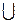C, where B and C are (Q,L)-fuzzy normal subsemiring of a semiring R, then either B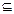C or CB.

Proof: It is trivial.

4. In the Following Theorem is the Composition Operation of Functions

4.1. Theorem 1

Let A be an (Q, L)-fuzzy normal subsemiring of a semiring H and f is an isomorphism from a semiring R onto H. Then A◦f is an (Q,L)-fuzzy normal subsemiring of the semiring R.

Proof: Let x and y in R and A be an (Q,L)-fuzzy normal subsemiring of a semiring H. Then clearly A◦f is an (Q,L)-fuzzy subsemiring of a semiring R. Now, (A◦f)( x+y, q) =A(f(x+y),q)=A(f(x)+f(y),q)=A(f(y)+f(x),q)=A(f(y+x),q) =(A◦f)(y+x,q),which implies that (A◦f)(x+y,q)=(A◦f)(y+x,q) , for all x and y in R and q in Q. And, (A◦f)(xy,q)= A(f(xy),q)=A(f(x)f(y),q)=A(f(y)f(x),q)=A(f(yx),q)= (A◦f)(yx,q), which implies that (A◦f)(xy,q)=(A◦f)(yx,q) , for all x and y in R and q in Q. Hence A◦f is an (Q,L)-fuzzy normal subsemiring of a semiring R.

4.2. Theorem 2

Let A be an (Q,L)-fuzzy normal subsemiring of a semiring H and f is an anti-isomorphism from a semiring R onto H. Then A◦f is an (Q,L)-fuzzy normal subsemiring of the semiring R.

Proof: Let x and y in R and A be an (Q,L)-fuzzy normal subsemiring of a semiring H. Then clearly A◦f is an (Q,L)-fuzzy subsemiring of a semiring R. Now, (A◦f)(x+y,q) = A(f(x+y),q)=A(f(y)+f(x),q)=A(f(x)+f(y),q)=A(f(y+x),q)= (A◦f)(y+x,q),which implies that (A◦f)(x+y,q)=(A◦f)(y+x,q) , for all x and y in R and q in Q. And, (A◦f)(xy,q) = A(f(xy),q)=A(f(y)f(x),q)=A(f(x)f(y),q)=A(f(yx),q)= (A◦f)(yx,q), which implies that (A◦f)(xy,q)=(A◦f)(yx,q) , for all x and y in R and q in Q. Hence A◦f is an (Q,L)-fuzzy normal subsemiring of a semiring R.

Acknowledgements

The authors would like to be thankful to the anonymous reviewers for their valuable suggestions.

References

1. Azriel Rosenfeld, Fuzzy Groups, Journal of mathematical analysis and applications, 35, 512-517 (1971).
2. Anthony. J. M. and Sherwood. H, Fuzzy groups Redefined, Journal of mathematical analysis and applications, 69,124 -130 (1979 ).
3. Asok Kumer Ray, On product of fuzzy subgroups, fuzzy sets and sysrems, 105, 181-183 (1999 ).
4. Biswas. R, Fuzzy subgroups and Anti-fuzzy subgroups, Fuzzy sets and systems, 35,121-124 ( 1990 ).
5. Mustafa Akgul, Some properties of fuzzy groups, Journal of mathematical analysis and applications, 133, 93-100 (1988).
6. Mohamed Asaad, Groups and fuzzy subgroups, fuzzy sets and systems (1991), North-Holland.
7. Palaniappan. N & Arjunan. K, Operation on fuzzy and anti fuzzy ideals, Antartica J. Math., 4(1) (2007), 59-64.
8. Prabir Bhattacharya, Fuzzy Subgroups: Some Characterizations, Journal of Mathematical Analysis and Applications, 128, 241-252 (1987).
9. Rajesh Kumar, Fuzzy Algebra, Volume 1, University of Delhi Publication Division, July -1993.
10. Salah Abou-Zaid, On generalized characteristic fuzzy subgroups of a finite group, fuzzy sets and systems, 235-241 (1991).
11. Sivaramakrishna das. P, Fuzzy groups and level subgroups, Journal of Mathematical Analysis and Applications, 84, 264-269 (1981).
12. Solairaju. A and Nagarajan. R, A New Structure and Construction of Q-Fuzzy Groups, Advances in fuzzy mathematics, Volume 4, Number 1 (2009), 23-29.
13. Tang J, Zhang X (2001). Product Operations in the Category of L –fuzzy groups. J. Fuzzy Math., 9:1-10.
14. Vasantha kandasamy. W. B, Smarandache fuzzy algebra, American research press, Rehoboth -2003.
15. Zadeh. L. A., Fuzzy sets , Information and control ,Vol.8, 338-353 (1965).

 Contents 1. 2. 2.1. 2.2. 2.3. 2.4. 2.5. 2.6. 2.7. 2.8. 2.9. 3. 3.1. 3.2. 3.3. 3.4. 3.5. 3.6. 3.7. 3.8. 3.9. 3.10. 3.11. 3.12. 3.13. 4. 4.1. 4.2.
Article ToolsAbstractPDF(177K)